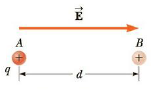Chapter 16, Problem 6P

Chapter
Section
Textbook Problem

A point charge q = +40.0 μC moves from A to B separated by a distance d = 0.180 m in the presence of an external electric field E → of magnitude 275 N/C directed toward the right as in Figure P16.6. Find (a) the electric force exerted on the charge, (b) the work done by the electric force, (c) the change in the electric potential energy of the charge, and (d) the potential difference between A and B.Figure P16.6

(a)

To determine
The electric force on the charge.

Explanation

Given info: The electric field strength (E) is 275 N/C. The charge (q) is 40.0μC . The distance (d) moved by the charge is 0.180 m.

Explanation:

Formula to calculate the electric force is,

F=qE

Substitute 40.0μC for q and 275 N/C for E.

F=(40.0μC)(275N/C)=(40

(b)

To determine
The work done by the force.

(c)

To determine
The change in electric potential energy.

(d)

To determine
The potential difference between A and B.

Still sussing out bartleby?

Check out a sample textbook solution.

See a sample solution

The Solution to Your Study Problems

Bartleby provides explanations to thousands of textbook problems written by our experts, many with advanced degrees!

Get Started

Find more solutions based on key concepts